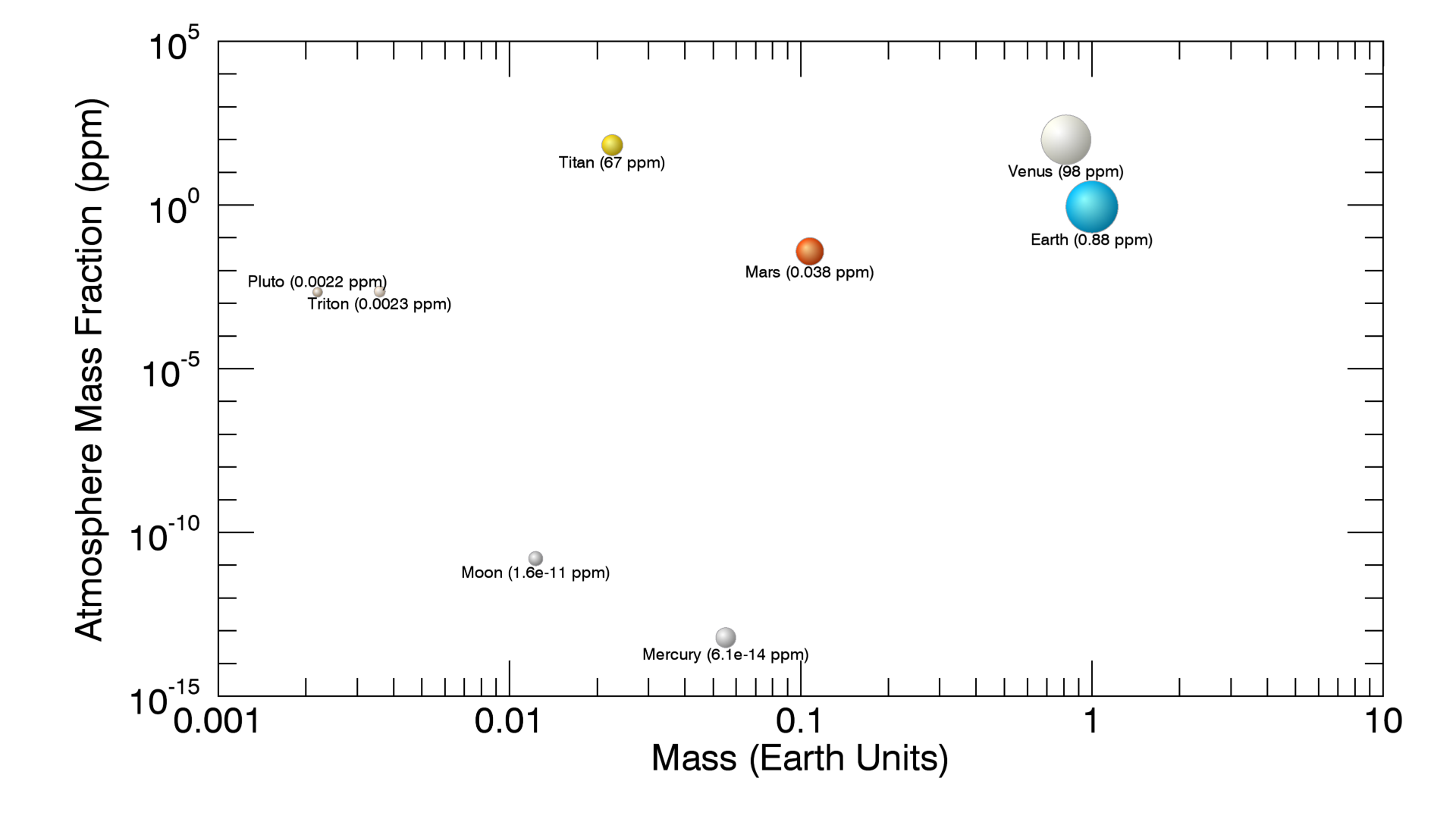Library‎ > ‎LabNotes‎ > ‎

### The Surface Pressure of Earth-like Exoplanets

posted Aug 18, 2011, 5:22 PM by Abel Mendez   [ updated Jan 24, 2016, 2:13 PM ]
 Earth's atmospheric mass is about 5.3 x 1018 kg, that is close to one part per million (ppm) of its total mass. The atmosphere mass fraction of a planet can be estimated from its radius, mass, and surface pressure. This is assuming that the atmosphere is small compared to the total planet's mass. The fraction of atmospheric to planet mass is given bywhere P is the mean surface pressure in bars, R is the radius and M the mass of the planet, both in Earth Units. The same equation can be used to get the fraction of each atmospheric component from their partial pressure. This fraction is shown for objects of the Solar System in Figure 1. The pressure of some gases in the atmosphere are limited by their condensation (e.g. water, carbon dioxide). As a reference, Figure 2 shows an atmospheric gas retention plot for hydrogen, nitrogen, and carbon dioxide.Figure 1. Atmosphere to planet mass fraction for Venus (98 ppm), Earth (0.9 ppm), Mars (0.04 ppm), Titan (67 ppm), Triton (0.002 ppm), and Pluto (0.002 ppm). The negligible atmospheres of the Moon and Mercury were also included for comparison purposes.Figure 2. Gas retention plot for hydrogen, nitrogen, and carbon dioxide for various Solar System objects. It shows escape velocity vs surface temperature. Earth and Venus are well within the hydrogen and nitrogen lines. Credit: University of Nebraska-Lincoln Astronomy Applets.    The equation can be used to estimate the potential surface pressure of Earth-like exoplanets given reasonable estimates of their atmosphere mass fraction. For example, Gliese 667Cc, has a minimum mass of 3.8 Earth masses which suggest a radius of 1.5 Earth radii assuming a rocky composition. If its atmosphere mass fraction is one ppm (just like Earth) then its surface pressure will be 3.3 bars. That is the pressure 23 meters below water, not that bad.Thanks to for pointing out the error in the original equation.